DraftKings World Series Odds
+600
6 to 1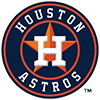Astros
11.6% implied probability

+700
7 to 1Yankees
10.2% implied probability

+750
7.5 to 1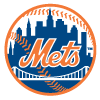Mets
9.6% implied probability

+800
8 to 1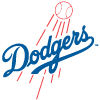Dodgers
9.1% implied probability

+950
9.5 to 1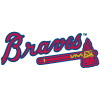Braves
7.8% implied probability

+1100
11 to 1Padres
6.8% implied probability

+1300
13 to 1Blue Jays
5.8% implied probability

+1700
17 to 1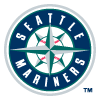Mariners
4.5% implied probability

+1800
18 to 1Phillies
4.3% implied probability

+1900
19 to 1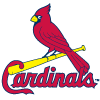Cardinals
4.1% implied probability

+2200
22 to 1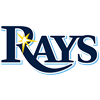Rays
3.5% implied probability

+2200
22 to 1Guardians
3.5% implied probability

+3000
30 to 1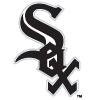White Sox
2.6% implied probability

+4000
40 to 1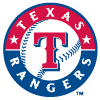Rangers
2.0% implied probability

+4000
40 to 1Brewers
2.0% implied probability

+4500
45 to 1Twins
1.8% implied probability

+5000
50 to 1Red Sox
1.6% implied probability

+5500
55 to 1Angels
1.5% implied probability

+6000
60 to 1Giants
1.3% implied probability

+7000
70 to 1Marlins
1.1% implied probability

+7000
70 to 1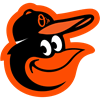Orioles
1.1% implied probability

+8000
80 to 1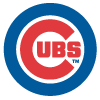Cubs
1.0% implied probability

+13000
130 to 1Diamondbacks
0.6% implied probability

+15000
150 to 1Tigers
0.5% implied probability

+20000
200 to 1Pirates
0.4% implied probability

+20000
200 to 1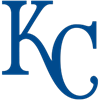Royals
0.4% implied probability

+20000
200 to 1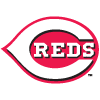Reds
0.4% implied probability

+25000
250 to 1Rockies
0.3% implied probability

+40000
400 to 1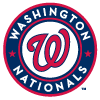Nationals
0.2% implied probability

+50000
500 to 1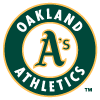Athletics
0.2% implied probability